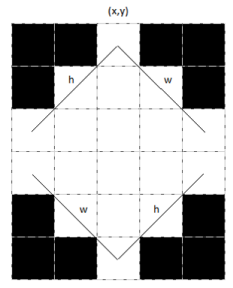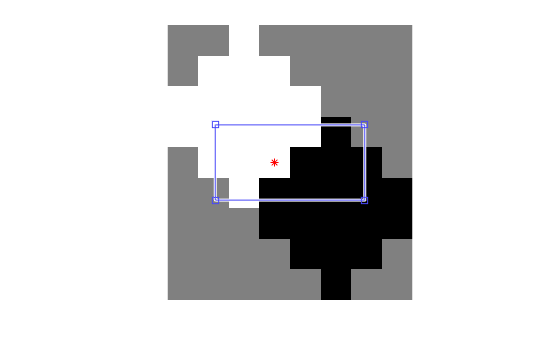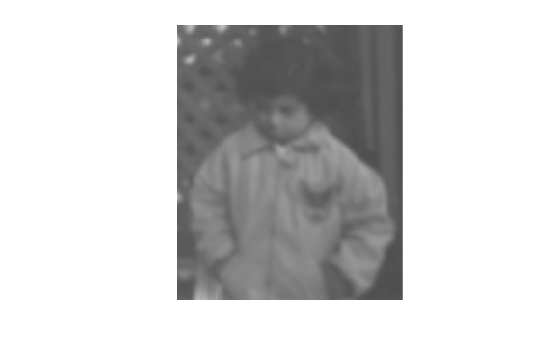# integralKernel

Define filter for use with integral images

## Description

An `integralKernel` object describes box filters for use with integral images.

## Creation

### Syntax

``intKernel = integralKernel(bbox,weights)``
``intKernel = integralKernel(bbox,weights,orientation)``

### Description

example

````intKernel = integralKernel(bbox,weights)` creates an upright box filter from bounding boxes, `bbox`, and their corresponding weights, `weights`. The bounding boxes set the BoundingBoxes property and the weights set the Weights property.For example, a conventional filter with the coefficients:and two regions:region 1: x=1, y=1, width = 4, height = 2region 2: x=1, y=3, width = 4, height = 2 can be specified asboxH = integralKernel([1 1 4 2; 1 3 4 2],[1, -1])```

example

````intKernel = integralKernel(bbox,weights,orientation)` creates a box filter with an upright or rotated orientation. The specified orientation sets the Orientation property.```

## Properties

expand all

Bounding boxes, specified as a 4-element vector of the form [x,y,width, height] representing a single bounding box or an M-by-4 matrix representing M bounding boxes. The bounding boxes define the filter. The (x,y) coordinates represent the top-most corner of the kernel. The (width, height) elements represent the width and height accordingly. Specifying the bounding boxes as an M-by-4 matrix is particularly useful for constructing Haar-like features composed of multiple rectangles.

Sums are computed over regions defined by `BoundingBoxes`. The bounding boxes can overlap. See Define an 11-by-11 Average Filter for an example of how to specify a box filter.

Weights, specified as an M-element numeric vector containing a weight for each bounding box. The weights are used to define the coefficients of the filter.

Filter coefficients, specified as a numeric value.

Filter center, specified as [x,y] coordinates. The filter center represents the center of the bounding rectangle. It is calculated by halving the dimensions of the rectangle. For even dimensional rectangles, the center is placed at subpixel locations. Hence, it is rounded up to the next integer.

For example, for this filter, the center is at [3,3].These coordinates are in the kernel space, where the top-left corner is (1,1). To place the center in a different location, provide the appropriate bounding box specification. For this filter, the best workflow would be to construct the upright kernel and then call the `rot45` method to provide the rotated version.

Filter size, specified as a 2-element vector. The size of the kernel is computed to be the dimensions of the rectangle that bounds the kernel. For a single bounding box vector [x,y,width, height], the kernel is bounded within a rectangle of dimensions [(width+height) (width+height)-1].

For cascaded rectangles, the lowest corner of the bottom-most rectangle defines the size. For example, a filter with a bounding box specification of [3 1 3 3], with weights set to 1, produces a 6-by-5 filter with this kernel:Filter orientation, specified as `'upright'` or `'rotated'`. When you specify the orientation as `'rotated'`, the (x,y) components refer to the location of the top-left corner of the bounding box. Also, the (width,height) components refer to a 45-degree line from the top-left corner of the bounding box.

## Usage

### Computing an Integral Image and Using it for Filtering with Box Filters

The `integralImage` function together with the `integralKernel` object and `integralFilter` function complete the workflow for box filtering based on integral images. You can use this workflow for filtering with box filters.

The `integralKernel` object allows you to transpose the filter. You can use this to aim a directional filter. For example, you can turn a horizontal edge detector into vertical edge detector.

## Object Functions

 `rot45` Rotate upright kernel clockwise by 45 degrees `transpose` Transpose integral kernel

## Examples

collapse all

` avgH = integralKernel([1 1 11 11], 1/11^2);`
`ydH = integralKernel([1,1,5,9;1,4,5,3], [1, -3]);`

You can also define this filter as `integralKernel`([1,1,5,3;1,4,5,3;1,7,5,3], [1, -2, 1]);|. This filter definition is less efficient because it requires three bounding boxes.

Visualize the filter.

`ydH.Coefficients`
```ans = 9×5 1 1 1 1 1 1 1 1 1 1 1 1 1 1 1 -2 -2 -2 -2 -2 -2 -2 -2 -2 -2 -2 -2 -2 -2 -2 1 1 1 1 1 1 1 1 1 1 1 1 1 1 1 ```

Create the filter.

`K = integralKernel([3,1,3,3;6 4 3 3], [1 -1], 'rotated');`

Visualize the filter and mark the center.

``` imshow(K.Coefficients, [], 'InitialMagnification', 'fit'); hold on; plot(K.Center(2),K.Center(1), 'r*'); impixelregion;```Read and display the input image.

``` I = imread('pout.tif'); imshow(I);```Compute the integral image.

` intImage = integralImage(I);`

Apply a 7-by-7 average filter.

``` avgH = integralKernel([1 1 7 7], 1/49); J = integralFilter(intImage, avgH);```

Cast the result back to the same class as the input image.

``` J = uint8(J); figure imshow(J);```Viola, Paul, and Michael J. Jones. “Rapid Object Detection using a Boosted Cascade of Simple Features”. Proceedings of the 2001 IEEE® Computer Society Conference on Computer Vision and Pattern Recognition. Vol. 1, 2001, pp. 511–518.